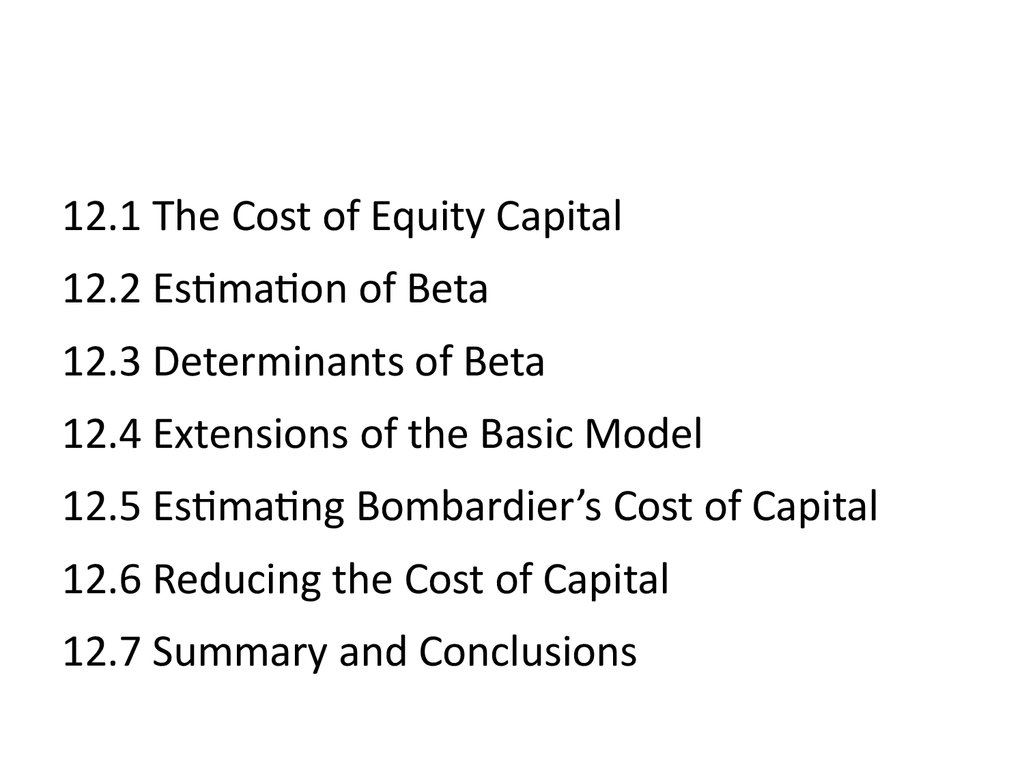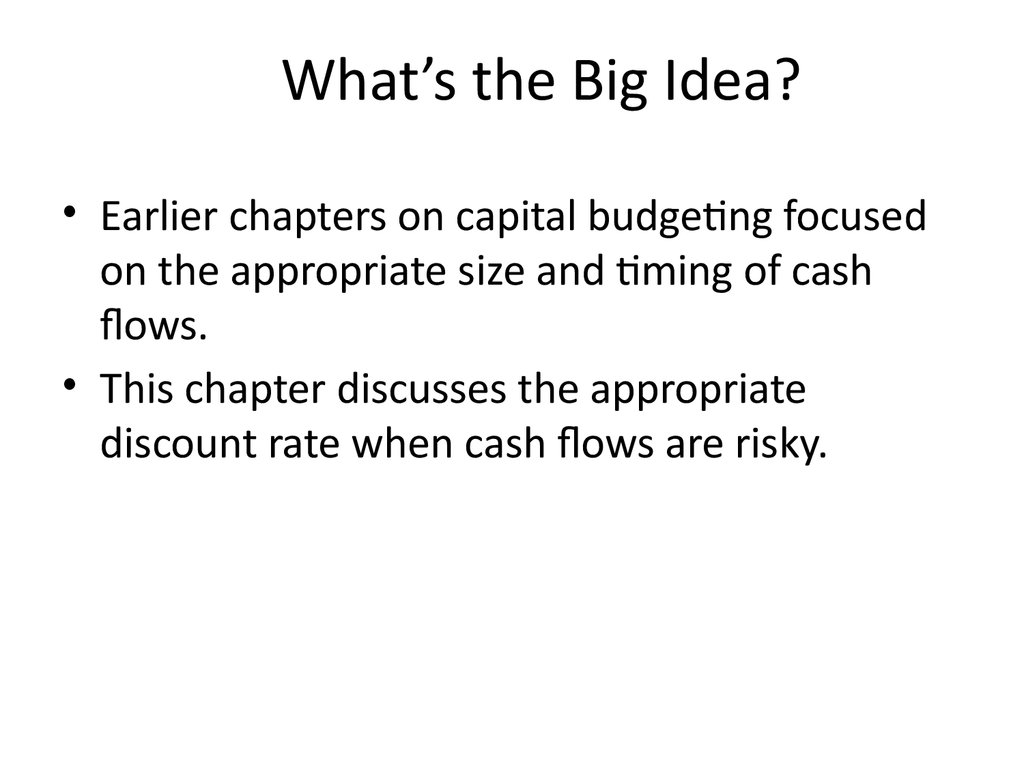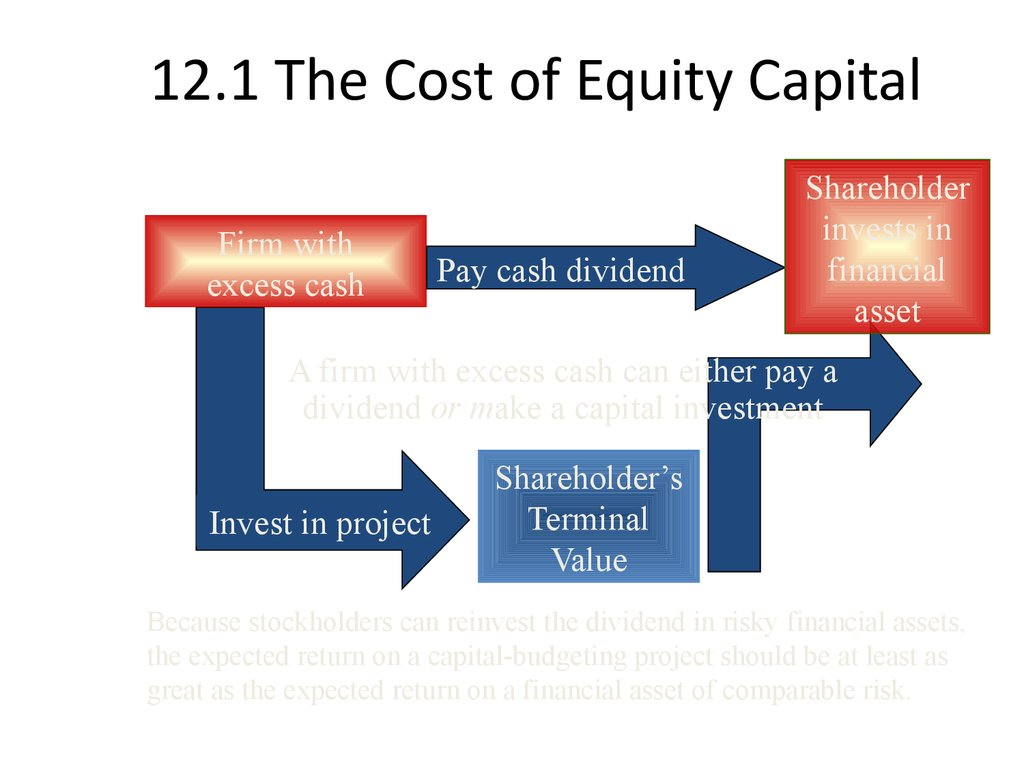# Risk Return and Project Decisions

## 1.

12.1 The Cost of Equity Capital
12.2 Estimation of Beta
12.3 Determinants of Beta
12.4 Extensions of the Basic Model
12.5 Estimating Bombardier’s Cost of Capital
12.6 Reducing the Cost of Capital
12.7 Summary and Conclusions

## 2. What’s the Big Idea?

• Earlier chapters on capital budgeting focused
on the appropriate size and timing of cash
flows.
• This chapter discusses the appropriate
discount rate when cash flows are risky.

## 3. 12.1 The Cost of Equity Capital

Firm with
excess cash
Pay cash dividend
Shareholder
invests in
financial
asset
A firm with excess cash can either pay a
dividend or make a capital investment
Invest in project
Shareholder’s
Terminal
Value
Because stockholders can reinvest the dividend in risky financial assets,
the expected return on a capital-budgeting project should be at least as
great as the expected return on a financial asset of comparable risk.

## 4. The Cost of Equity

• From the firm’s perspective, the expected return
is the Cost of Equity Capital:
R i RF βi ( R M RF )
• To estimate a firm’s cost of equity capital, we need
to know three things:
1. The risk-free rate, RF
2. The market risk premium, R
R
M
F
Cov
(
R
R
) σ
i
,
M
i,
M
2
3. The company beta, β
i
V(
ar
R
) σ
M
M

## 5. Example

• Suppose the stock of Stansfield Enterprises, a
publisher of PowerPoint presentations, has a beta
of 2.5. The firm is 100-percent equity financed.
• Assume a risk-free rate of 5-percent and a market
• What is the appropriate discount rate for an
expansion of this firm?
R RF βi ( R M RF )
R 5% 2.5 10%
R 30%

## 6. Example (continued)

Suppose Stansfield Enterprises is evaluating
the following non-mutually exclusive
projects. Each costs \$100 and lasts one year.
Project
Project b
A
IRR
NPV at
30%
2.5
Project’s
Estimated Cash
Flows Next
Year
\$150
50%
\$15.38
B
2.5
\$130
30%
\$0
C
2.5
\$110
10%
-\$15.38

## 7. Using the SML to Estimate the Risk-Adjusted Discount Rate for Projects

IRR
Project
Using the SML to Estimate the RiskAdjusted Discount Rate for Projects
Good
A
projects
30%
B
5%
C
SML
Firm’s risk (beta)
2.5
An all-equity firm should accept a project whose IRR exceeds the cost
of equity capital and reject projects whose IRRs fall short of the cost
of capital.

## 8. 12.2 Estimation of Beta: Measuring Market Risk

Market Portfolio - Portfolio of all assets in the
economy. In practice a broad stock market
index, such as the TSE 300 index, is used to
represent the market.
return on the market portfolio.

## 9. 12.2 Estimation of Beta

• Theoretically, the calculation of beta is straightforward:
Problems
Cov( Ri , RM ) σ i2
β
2
Var ( RM )
σM
1. Betas may vary over time.
2. The sample size may be inadequate.
3. Betas are influenced by changing financial leverage and business
risk.
Solutions
– Problems 1 and 2 (above) can be moderated by more
sophisticated statistical techniques.
– Problem 3 can be lessened by adjusting for changes in business
and financial risk.
– Look at average beta estimates of comparable firms in the
industry.

## 10. Stability of Beta

• Most analysts argue that betas are generally stable
for firms remaining in the same industry.
• That’s not to say that a firm’s beta can’t change.
– Changes in product line
– Changes in technology
– Deregulation
– Changes in financial leverage

## 11. Using an Industry Beta

• It is frequently argued that one can better estimate a
firm’s beta by involving the whole industry.
• If you believe that the operations of the firm are
similar to the operations of the rest of the industry,
you should use the industry beta.
• If you believe that the operations of the firm are
fundamentally different from the operations of the
rest of the industry, you should use the firm’s beta.

## 12. 12.3 Determinants of Beta

– Cyclicity of Revenues
– Operating Leverage
• Financial Risk
– Financial Leverage

## 13. Cyclicality of Revenues

• Highly cyclical stocks have high betas.
– Empirical evidence suggests that retailers and
automotive firms fluctuate with the business cycle.
– Transportation firms and utilities are less dependent
• Note that cyclicality is not the same as variability—
stocks with high standard deviations need not have
high betas.
– Movie studios have revenues that are variable,
depending upon whether they produce “hits” or
“flops,” but their revenues are not especially

## 14. Operating Leverage

• The degree of operating leverage measures how
sensitive a firm (or project) is to its fixed costs.
• Operating leverage increases as fixed costs rise and
variable costs fall.
• Operating leverage magnifies the effect of cyclicity
on beta.
• The degree of operating leverage is given by:
Change in EBIT
Sales
DOL
EBIT
Change in Sales

## 15. Operating Leverage

\$
Total
costs
Fixed costs
EBIT
Volume
Fixed costs
Volume
Operating leverage increases as fixed costs rise
and variable costs fall.

## 16. Financial Leverage and Beta

• Operating leverage refers to the sensitivity to the firm’s
fixed costs of production.
• Financial leverage is the sensitivity of a firm’s fixed costs
of financing.
• The relationship between the betas of the firm’s debt,
equity, and assets is given by:
β Asset
Debt
Equity
βDebt
βEquity
Debt Equity
Debt Equity
• Financial leverage always increases the equity beta relative
to the asset beta.

## 17. Financial Leverage and Beta: Example

Consider Grand Sport, Inc., which is currently all-equity
and has a beta of 0.90.
The firm has decided to lever up to a capital structure
of 1 part debt to 1 part equity.
Since the firm will remain in the same industry, its asset
beta should remain 0.90.
However, assuming a zero beta for its debt, its equity
beta would become twice as large:
βEquity
Debt
1
0.90 1 1.80
βAsset 1
1
Equity

## 18. 12.4 Extensions of the Basic Model

• The Firm versus the Project
• The Cost of Capital with Debt

## 19. The Firm versus the Project

• Any project’s cost of capital depends on the use to
which the capital is being put—not the source.
• Therefore, it depends on the risk of the project and
not the risk of the company.

## 20. Capital Budgeting & Project Risk

Project
IRR
Capital Budgeting & Project Risk
The SML can tell us why:
SML
Incorrectly accepted
negative NPV projects
RF βFIRM ( R M RF )
Hurdle
rate
rf
bFIRM
Incorrectly rejected
positive NPV projects
Firm’s risk (beta)
A firm that uses one discount rate for all projects may over
time increase the risk of the firm while decreasing its value.

## 21. Capital Budgeting & Project Risk

Capital Budgeting & Project Risk
Suppose the Conglomerate Company has a cost of
capital, based on the CAPM, of 17%. The risk-free
rate is 4%, the market risk premium is 10%, and
the firm’s beta is 1.3.
17% = 4% + 1.3 × [14% – 4%]
retailer
b = 2.0
This1/3
is Automotive
a breakdown
of the
company’s investment
1/3 Computer Hard Drive Mfr. b = 1.3
projects:
1/3 Electric Utility b = 0.6
average b of assets = 1.3
When evaluating a new electrical generation investment,
which cost of capital should be used?

## 22. Capital Budgeting & Project Risk

SML
IRR
Project
Capital Budgeting & Project Risk
24%
Investments in hard
drives or auto retailing
should have higher
discount rates.
17%
10%
Firm’s risk (beta)
0.6
1.3
2.0
r = 4% + 0.6×(14% – 4% ) = 10%
10% reflects the opportunity cost of capital on an investment in
electrical generation, given the unique risk of the project.

## 23. The Cost of Capital with Debt

• The Weighted Average Cost of Capital is given by:
rWACC
S
B
rS
rB (1 TC )
S B
S B
• It is because interest expense is tax-deductible that
we multiply the last term by (1- TC)

## 24. 12.5 Estimating Bombardier’s Cost of Capital

• We aim at estimating Bombardier’s cost of capital, as of
June 15, 2001.
• First, we estimate the cost of equity and the cost of debt.
– We estimate an equity beta to estimate the cost of
equity.
– We can often estimate the cost of debt by observing
the YTM of the firm’s debt.
• Second, we determine the WACC by weighting these two
costs appropriately.

## 25. 12.5 Estimating Bombardier’s Cost of Capital

• Bombardier’s beta is 0.79; the (current) risk-free rate
is 4.07%, and the (historical) market risk premium is
6.89%.
• Thus the cost of equity capital is
re RF βi ( R M RF )
4.07% 0.79 6.89%
9.51%

## 26. 12.5 Estimating Bombardier’s Cost of Capital

• The yield on the company’s 6.6% 29 Nov 04 bond
is 5.73% and the firm is in the 40% marginal tax
rate.
• Thus the cost of debt is
rB (1 TC ) 5.73% (1 0.40) 3.44%

## 27.

12.5 Estimating Bombardier’s Cost of Capital
• To calculate the cost of capital, we need to
estimate the value weights for equity and debt:
Market Value Weights for Bombardier (as of January 31, 2001)
Security
Debt (book value)
Preferred stock
Class A Common
Class B Common
Market
Price
25.75
23.09
23.27
Shares
Market Value
Outstanding
(millions) Weight (%)
\$
6,131
16.07%
12,000,000 \$
309
0.81%
347,426,000 \$
8,022
21.02%
1,018,625,000 \$
23,703
62.10%
Total
3816547%
100.00%
• We simplify, and combine preferred stock with
common stock:
S
B
83.93%, and
16.07%
S B
S B

## 28.

12.5 Estimating Bombardier’s Cost of Capital
• Bombardier’s WACC as of June 15, 2001, is given
by:
S
B
rWACC
r
S
rB (1 TC )
S B
S B
0.8393 9.51% 0.1607 5.73% (1 0.4)
8.53%
8.53-percent is Bombardier’s cost of capital. It should be used
to discount any project where one believes that the project’s
risk is equal to the risk of the firm as a whole, and the project
has the same leverage as the firm as a whole.

## 29. 12.6 Reducing the Cost of Capital

What is Liquidity?
Liquidity, Expected Returns, and the Cost of Capital
What the Corporation Can Do

## 30. What is Liquidity?

• The idea that the expected return on a stock and the
firm’s cost of capital are positively related to risk is
fundamental.
• Recently a number of academics have argued that
the expected return on a stock and the firm’s cost of
capital are negatively related to the liquidity of the
firm’s shares as well.
• The trading costs of holding a firm’s shares include
impact costs.

## 31. Liquidity, Expected Returns, and the Cost of Capital

• The cost of trading an illiquid stock reduces the total
• Investors thus will demand a high expected return
when investing in stocks with high trading costs.
• This high expected return implies a high cost of
capital to the firm.

## 32. Liquidity and the Cost of Capital

Cost of Capital
Liquidity and the Cost of Capital
Liquidity
An increase in liquidity, i.e., a reduction in trading costs,
lowers a firm’s cost of capital.

## 33. Liquidity and Adverse Selection

• There are a number of factors that determine the
liquidity of a stock.
• One of these factors is adverse selection.
• This refers to the notion that traders with better
information can take advantage of specialists and
other traders who have less information.
• The greater the heterogeneity of information, the
required return on equity.

## 34. What the Corporation Can Do

• The corporation has an incentive to lower trading
costs since this would result in a lower cost of capital.
• A stock split would increase the liquidity of the
shares.
• A stock split would also reduce the adverse selection
• This idea is a new one and empirical evidence is not
yet in.

## 35. What the Corporation Can Do

• Companies can also facilitate stock purchases
through the Internet.
• Direct stock purchase plans and dividend
reinvestment plans handled on-line allow small
investors the opportunity to buy securities cheaply.
especially to security analysts, to narrow the gap
between informed and uninformed traders. This

## 36. 12.7 Summary and Conclusions

• The expected return on any capital budgeting project
should be at least as great as the expected return on a
financial asset of comparable risk. Otherwise the
shareholders would prefer the firm to pay a dividend.
• The expected return on any asset is dependent upon b.
• A project’s required return depends on the project’s b.
• A project’s b can be estimated by considering
comparable industries or the cyclicality of project
revenues and the project’s operating leverage.
• If the firm uses debt, the discount rate to use is the rWACC.
• In order to calculate rWACC, the cost of equity and the cost
of debt applicable to a project must be estimated.